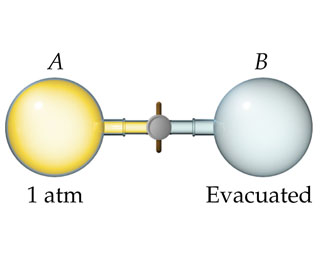# Problem: Consider a system consisting of the following apparatus, in which gas is confined in one flask and there is a vacuum in the other flask. The flasks are separated by a valve. Assume that the flasks are perfectly insulated and will not allow the flow of heat into or out of the flasks to the surroundings. When the valve is opened, gas flows from the filled flask to the evacuated one.Is work performed during the expansion of the gas? Why or why not?

###### FREE Expert Solution

Recall that work (w) is given by:

$\overline{){\mathbf{w}}{\mathbf{=}}{\mathbf{-}}{\mathbf{P\Delta V}}}$

Internal Energy, U:

$\mathbf{U}\mathbf{=}\mathbf{q}\mathbf{+}\mathbf{w}$

93% (376 ratings)###### Problem Details

Consider a system consisting of the following apparatus, in which gas is confined in one flask and there is a vacuum in the other flask. The flasks are separated by a valve. Assume that the flasks are perfectly insulated and will not allow the flow of heat into or out of the flasks to the surroundings. When the valve is opened, gas flows from the filled flask to the evacuated one.Is work performed during the expansion of the gas? Why or why not?